# QRect Class

The QRect class defines a rectangle in the plane using integer precision. More...

 Header: #include qmake: QT += core

Note: All functions in this class are reentrant.

## Public Functions

 QRect() QRect(const QPoint &topLeft, const QPoint &bottomRight) QRect(const QPoint &topLeft, const QSize &size) QRect(int x, int y, int width, int height) void adjust(int dx1, int dy1, int dx2, int dy2) QRect adjusted(int dx1, int dy1, int dx2, int dy2) const int bottom() const QPoint bottomLeft() const QPoint bottomRight() const QPoint center() const bool contains(const QPoint &point, bool proper = false) const bool contains(const QRect &rectangle, bool proper = false) const bool contains(int x, int y) const bool contains(int x, int y, bool proper) const void getCoords(int *x1, int *y1, int *x2, int *y2) const void getRect(int *x, int *y, int *width, int *height) const int height() const QRect intersected(const QRect &rectangle) const bool intersects(const QRect &rectangle) const bool isEmpty() const bool isNull() const bool isValid() const int left() const QRect marginsAdded(const QMargins &margins) const QRect marginsRemoved(const QMargins &margins) const void moveBottom(int y) void moveBottomLeft(const QPoint &position) void moveBottomRight(const QPoint &position) void moveCenter(const QPoint &position) void moveLeft(int x) void moveRight(int x) void moveTo(int x, int y) void moveTo(const QPoint &position) void moveTop(int y) void moveTopLeft(const QPoint &position) void moveTopRight(const QPoint &position) QRect normalized() const int right() const void setBottom(int y) void setBottomLeft(const QPoint &position) void setBottomRight(const QPoint &position) void setCoords(int x1, int y1, int x2, int y2) void setHeight(int height) void setLeft(int x) void setRect(int x, int y, int width, int height) void setRight(int x) void setSize(const QSize &size) void setTop(int y) void setTopLeft(const QPoint &position) void setTopRight(const QPoint &position) void setWidth(int width) void setX(int x) void setY(int y) QSize size() const CGRect toCGRect() const int top() const QPoint topLeft() const QPoint topRight() const void translate(int dx, int dy) void translate(const QPoint &offset) QRect translated(int dx, int dy) const QRect translated(const QPoint &offset) const QRect transposed() const QRect united(const QRect &rectangle) const int width() const int x() const int y() const QRect operator&(const QRect &rectangle) const QRect & operator&=(const QRect &rectangle) QRect & operator+=(const QMargins &margins) QRect & operator-=(const QMargins &margins) QRect operator|(const QRect &rectangle) const QRect & operator|=(const QRect &rectangle)
 bool operator!=(const QRect &r1, const QRect &r2) QRect operator+(const QRect &rectangle, const QMargins &margins) QRect operator+(const QMargins &margins, const QRect &rectangle) QRect operator-(const QRect &lhs, const QMargins &rhs) QDataStream & operator<<(QDataStream &stream, const QRect &rectangle) bool operator==(const QRect &r1, const QRect &r2) QDataStream & operator>>(QDataStream &stream, QRect &rectangle)

## Detailed Description

The QRect class defines a rectangle in the plane using integer precision.

A rectangle is normally expressed as a top-left corner and a size. The size (width and height) of a QRect is always equivalent to the mathematical rectangle that forms the basis for its rendering.

A QRect can be constructed with a set of left, top, width and height integers, or from a QPoint and a QSize. The following code creates two identical rectangles.

```QRect r1(100, 200, 11, 16);
QRect r2(QPoint(100, 200), QSize(11, 16));```

There is a third constructor that creates a QRect using the top-left and bottom-right coordinates, but we recommend that you avoid using it. The rationale is that for historical reasons the values returned by the bottom() and right() functions deviate from the true bottom-right corner of the rectangle.

The QRect class provides a collection of functions that return the various rectangle coordinates, and enable manipulation of these. QRect also provides functions to move the rectangle relative to the various coordinates. In addition there is a moveTo() function that moves the rectangle, leaving its top left corner at the given coordinates. Alternatively, the translate() function moves the rectangle the given offset relative to the current position, and the translated() function returns a translated copy of this rectangle.

The size() function returns the rectange's dimensions as a QSize. The dimensions can also be retrieved separately using the width() and height() functions. To manipulate the dimensions use the setSize(), setWidth() or setHeight() functions. Alternatively, the size can be changed by applying either of the functions setting the rectangle coordinates, for example, setBottom() or setRight().

The contains() function tells whether a given point is inside the rectangle or not, and the intersects() function returns `true` if this rectangle intersects with a given rectangle. The QRect class also provides the intersected() function which returns the intersection rectangle, and the united() function which returns the rectangle that encloses the given rectangle and this: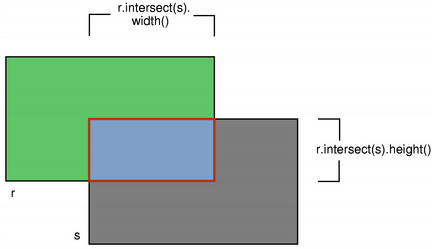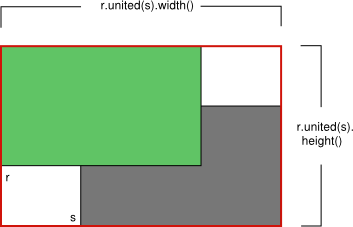intersected() united()

The isEmpty() function returns `true` if left() > right() or top() > bottom(). Note that an empty rectangle is not valid: The isValid() function returns `true` if left() <= right() and top() <= bottom(). A null rectangle (isNull() == true) on the other hand, has both width and height set to 0.

Note that due to the way QRect and QRectF are defined, an empty QRect is defined in essentially the same way as QRectF.

Finally, QRect objects can be streamed as well as compared.

### Rendering

When using an anti-aliased painter, the boundary line of a QRect will be rendered symmetrically on both sides of the mathematical rectangle's boundary line. But when using an aliased painter (the default) other rules apply.

Then, when rendering with a one pixel wide pen the QRect's boundary line will be rendered to the right and below the mathematical rectangle's boundary line.

When rendering with a two pixels wide pen the boundary line will be split in the middle by the mathematical rectangle. This will be the case whenever the pen is set to an even number of pixels, while rendering with a pen with an odd number of pixels, the spare pixel will be rendered to the right and below the mathematical rectangle as in the one pixel case.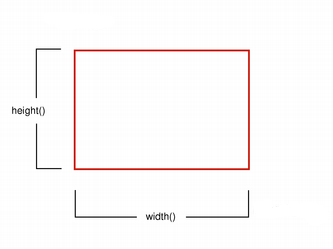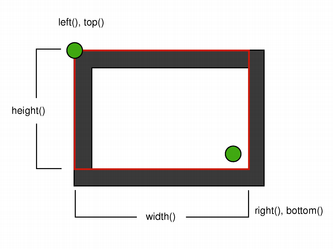Logical representation One pixel wide pen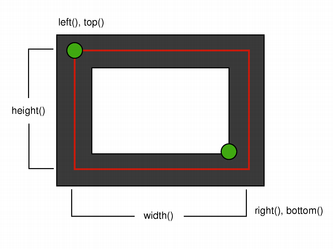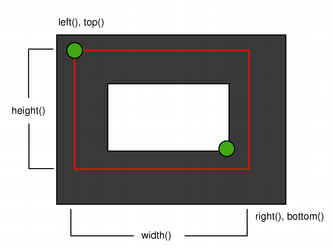Two pixel wide pen Three pixel wide pen

### Coordinates

The QRect class provides a collection of functions that return the various rectangle coordinates, and enable manipulation of these. QRect also provides functions to move the rectangle relative to the various coordinates.

For example the left(), setLeft() and moveLeft() functions as an example: left() returns the x-coordinate of the rectangle's left edge, setLeft() sets the left edge of the rectangle to the given x coordinate (it may change the width, but will never change the rectangle's right edge) and moveLeft() moves the entire rectangle horizontally, leaving the rectangle's left edge at the given x coordinate and its size unchanged.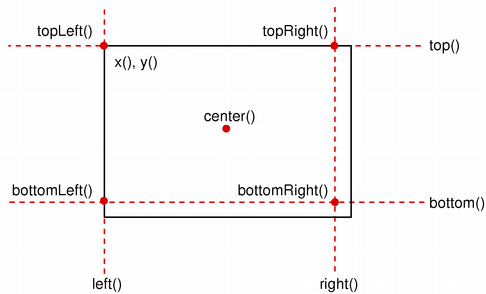Note that for historical reasons the values returned by the bottom() and right() functions deviate from the true bottom-right corner of the rectangle: The right() function returns left() + width() - 1 and the bottom() function returns top() + height() - 1. The same is the case for the point returned by the bottomRight() convenience function. In addition, the x and y coordinate of the topRight() and bottomLeft() functions, respectively, contain the same deviation from the true right and bottom edges.

We recommend that you use x() + width() and y() + height() to find the true bottom-right corner, and avoid right() and bottom(). Another solution is to use QRectF: The QRectF class defines a rectangle in the plane using floating point accuracy for coordinates, and the QRectF::right() and QRectF::bottom() functions do return the right and bottom coordinates.

It is also possible to add offsets to this rectangle's coordinates using the adjust() function, as well as retrieve a new rectangle based on adjustments of the original one using the adjusted() function. If either of the width and height is negative, use the normalized() function to retrieve a rectangle where the corners are swapped.

In addition, QRect provides the getCoords() function which extracts the position of the rectangle's top-left and bottom-right corner, and the getRect() function which extracts the rectangle's top-left corner, width and height. Use the setCoords() and setRect() function to manipulate the rectangle's coordinates and dimensions in one go.

### Constraints

QRect is limited to the minimum and maximum values for the `int` type. Operations on a QRect that could potentially result in values outside this range will result in undefined behavior.

## Member Function Documentation

### QRect::QRect()

Constructs a null rectangle.

### QRect::QRect(const QPoint &topLeft, const QPoint &bottomRight)

Constructs a rectangle with the given topLeft and bottomRight corners.

### QRect::QRect(const QPoint &topLeft, const QSize &size)

Constructs a rectangle with the given topLeft corner and the given size.

### QRect::QRect(intx, inty, intwidth, intheight)

Constructs a rectangle with (x, y) as its top-left corner and the given width and height.

### void QRect::adjust(intdx1, intdy1, intdx2, intdy2)

Adds dx1, dy1, dx2 and dy2 respectively to the existing coordinates of the rectangle.

### QRect QRect::adjusted(intdx1, intdy1, intdx2, intdy2) const

Returns a new rectangle with dx1, dy1, dx2 and dy2 added respectively to the existing coordinates of this rectangle.

### int QRect::bottom() const

Returns the y-coordinate of the rectangle's bottom edge.

Note that for historical reasons this function returns top() + height() - 1; use y() + height() to retrieve the true y-coordinate.

### QPoint QRect::bottomLeft() const

Returns the position of the rectangle's bottom-left corner. Note that for historical reasons this function returns QPoint(left(), top() + height() - 1).

### QPoint QRect::bottomRight() const

Returns the position of the rectangle's bottom-right corner.

Note that for historical reasons this function returns QPoint(left() + width() -1, top() + height() - 1).

### QPoint QRect::center() const

Returns the center point of the rectangle.

### bool QRect::contains(const QPoint &point, boolproper = false) const

Returns `true` if the given point is inside or on the edge of the rectangle, otherwise returns `false`. If proper is true, this function only returns `true` if the given point is inside the rectangle (i.e., not on the edge).

### bool QRect::contains(const QRect &rectangle, boolproper = false) const

Returns `true` if the given rectangle is inside this rectangle. otherwise returns `false`. If proper is true, this function only returns `true` if the rectangle is entirely inside this rectangle (not on the edge).

### bool QRect::contains(intx, inty) const

Returns `true` if the point (x, y) is inside this rectangle, otherwise returns `false`.

### bool QRect::contains(intx, inty, boolproper) const

Returns `true` if the point (x, y) is inside or on the edge of the rectangle, otherwise returns `false`. If proper is true, this function only returns `true` if the point is entirely inside the rectangle(not on the edge).

### void QRect::getCoords(int *x1, int *y1, int *x2, int *y2) const

Extracts the position of the rectangle's top-left corner to *x1 and *y1, and the position of the bottom-right corner to *x2 and *y2.

### void QRect::getRect(int *x, int *y, int *width, int *height) const

Extracts the position of the rectangle's top-left corner to *x and *y, and its dimensions to *width and *height.

### int QRect::height() const

Returns the height of the rectangle.

### QRect QRect::intersected(const QRect &rectangle) const

Returns the intersection of this rectangle and the given rectangle. Note that `r.intersected(s)` is equivalent to `r & s`.This function was introduced in Qt 4.2.

### bool QRect::intersects(const QRect &rectangle) const

Returns `true` if this rectangle intersects with the given rectangle (i.e., there is at least one pixel that is within both rectangles), otherwise returns `false`.

The intersection rectangle can be retrieved using the intersected() function.

### bool QRect::isEmpty() const

Returns `true` if the rectangle is empty, otherwise returns `false`.

An empty rectangle has a left() > right() or top() > bottom(). An empty rectangle is not valid (i.e., isEmpty() == !isValid()).

Use the normalized() function to retrieve a rectangle where the corners are swapped.

### bool QRect::isNull() const

Returns `true` if the rectangle is a null rectangle, otherwise returns `false`.

A null rectangle has both the width and the height set to 0 (i.e., right() == left() - 1 and bottom() == top() - 1). A null rectangle is also empty, and hence is not valid.

### bool QRect::isValid() const

Returns `true` if the rectangle is valid, otherwise returns `false`.

A valid rectangle has a left() <= right() and top() <= bottom(). Note that non-trivial operations like intersections are not defined for invalid rectangles. A valid rectangle is not empty (i.e., isValid() == !isEmpty()).

### int QRect::left() const

Returns the x-coordinate of the rectangle's left edge. Equivalent to x().

### QRect QRect::marginsAdded(const QMargins &margins) const

Returns a rectangle grown by the margins.

This function was introduced in Qt 5.1.

### QRect QRect::marginsRemoved(const QMargins &margins) const

Removes the margins from the rectangle, shrinking it.

This function was introduced in Qt 5.1.

### void QRect::moveBottom(inty)

Moves the rectangle vertically, leaving the rectangle's bottom edge at the given y coordinate. The rectangle's size is unchanged.

### void QRect::moveBottomLeft(const QPoint &position)

Moves the rectangle, leaving the bottom-left corner at the given position. The rectangle's size is unchanged.

### void QRect::moveBottomRight(const QPoint &position)

Moves the rectangle, leaving the bottom-right corner at the given position. The rectangle's size is unchanged.

### void QRect::moveCenter(const QPoint &position)

Moves the rectangle, leaving the center point at the given position. The rectangle's size is unchanged.

### void QRect::moveLeft(intx)

Moves the rectangle horizontally, leaving the rectangle's left edge at the given x coordinate. The rectangle's size is unchanged.

### void QRect::moveRight(intx)

Moves the rectangle horizontally, leaving the rectangle's right edge at the given x coordinate. The rectangle's size is unchanged.

### void QRect::moveTo(intx, inty)

Moves the rectangle, leaving the top-left corner at the given position (x, y). The rectangle's size is unchanged.

### void QRect::moveTo(const QPoint &position)

Moves the rectangle, leaving the top-left corner at the given position.

### void QRect::moveTop(inty)

Moves the rectangle vertically, leaving the rectangle's top edge at the given y coordinate. The rectangle's size is unchanged.

### void QRect::moveTopLeft(const QPoint &position)

Moves the rectangle, leaving the top-left corner at the given position. The rectangle's size is unchanged.

### void QRect::moveTopRight(const QPoint &position)

Moves the rectangle, leaving the top-right corner at the given position. The rectangle's size is unchanged.

### QRect QRect::normalized() const

Returns a normalized rectangle; i.e., a rectangle that has a non-negative width and height.

If width() < 0 the function swaps the left and right corners, and it swaps the top and bottom corners if height() < 0.

Returns the x-coordinate of the rectangle's right edge.

Note that for historical reasons this function returns left() + width() - 1; use x() + width() to retrieve the true x-coordinate.

### void QRect::setBottom(inty)

Sets the bottom edge of the rectangle to the given y coordinate. May change the height, but will never change the top edge of the rectangle.

### void QRect::setBottomLeft(const QPoint &position)

Set the bottom-left corner of the rectangle to the given position. May change the size, but will never change the top-right corner of the rectangle.

### void QRect::setBottomRight(const QPoint &position)

Set the bottom-right corner of the rectangle to the given position. May change the size, but will never change the top-left corner of the rectangle.

### void QRect::setCoords(intx1, inty1, intx2, inty2)

Sets the coordinates of the rectangle's top-left corner to (x1, y1), and the coordinates of its bottom-right corner to (x2, y2).

### void QRect::setHeight(intheight)

Sets the height of the rectangle to the given height. The bottom edge is changed, but not the top one.

### void QRect::setLeft(intx)

Sets the left edge of the rectangle to the given x coordinate. May change the width, but will never change the right edge of the rectangle.

Equivalent to setX().

### void QRect::setRect(intx, inty, intwidth, intheight)

Sets the coordinates of the rectangle's top-left corner to (x, y), and its size to the given width and height.

### void QRect::setRight(intx)

Sets the right edge of the rectangle to the given x coordinate. May change the width, but will never change the left edge of the rectangle.

### void QRect::setSize(const QSize &size)

Sets the size of the rectangle to the given size. The top-left corner is not moved.

### void QRect::setTop(inty)

Sets the top edge of the rectangle to the given y coordinate. May change the height, but will never change the bottom edge of the rectangle.

Equivalent to setY().

### void QRect::setTopLeft(const QPoint &position)

Set the top-left corner of the rectangle to the given position. May change the size, but will never change the bottom-right corner of the rectangle.

### void QRect::setTopRight(const QPoint &position)

Set the top-right corner of the rectangle to the given position. May change the size, but will never change the bottom-left corner of the rectangle.

### void QRect::setWidth(intwidth)

Sets the width of the rectangle to the given width. The right edge is changed, but not the left one.

### void QRect::setX(intx)

Sets the left edge of the rectangle to the given x coordinate. May change the width, but will never change the right edge of the rectangle.

Equivalent to setLeft().

### void QRect::setY(inty)

Sets the top edge of the rectangle to the given y coordinate. May change the height, but will never change the bottom edge of the rectangle.

Equivalent to setTop().

### QSize QRect::size() const

Returns the size of the rectangle.

### CGRect QRect::toCGRect() const

Creates a CGRect from a QRect.

This function was introduced in Qt 5.8.

### int QRect::top() const

Returns the y-coordinate of the rectangle's top edge. Equivalent to y().

### QPoint QRect::topLeft() const

Returns the position of the rectangle's top-left corner.

### QPoint QRect::topRight() const

Returns the position of the rectangle's top-right corner.

Note that for historical reasons this function returns QPoint(left() + width() -1, top()).

### void QRect::translate(intdx, intdy)

Moves the rectangle dx along the x axis and dy along the y axis, relative to the current position. Positive values move the rectangle to the right and down.

### void QRect::translate(const QPoint &offset)

Moves the rectangle offset.x() along the x axis and offset.y() along the y axis, relative to the current position.

### QRect QRect::translated(intdx, intdy) const

Returns a copy of the rectangle that is translated dx along the x axis and dy along the y axis, relative to the current position. Positive values move the rectangle to the right and down.

### QRect QRect::translated(const QPoint &offset) const

Returns a copy of the rectangle that is translated offset.x() along the x axis and offset.y() along the y axis, relative to the current position.

### QRect QRect::transposed() const

Returns a copy of the rectangle that has its width and height exchanged:

```QRect r = {15, 51, 42, 24};
r = r.transposed(); // r == {15, 51, 24, 42}```

This function was introduced in Qt 5.7.

### QRect QRect::united(const QRect &rectangle) const

Returns the bounding rectangle of this rectangle and the given rectangle.This function was introduced in Qt 4.2.

### int QRect::width() const

Returns the width of the rectangle.

### int QRect::x() const

Returns the x-coordinate of the rectangle's left edge. Equivalent to left().

### int QRect::y() const

Returns the y-coordinate of the rectangle's top edge. Equivalent to top().

### QRect QRect::operator&(const QRect &rectangle) const

Returns the intersection of this rectangle and the given rectangle. Returns an empty rectangle if there is no intersection.

### QRect &QRect::operator&=(const QRect &rectangle)

Intersects this rectangle with the given rectangle.

### QRect &QRect::operator+=(const QMargins &margins)

Adds the margins to the rectangle, growing it.

This function was introduced in Qt 5.1.

### QRect &QRect::operator-=(const QMargins &margins)

Returns a rectangle shrunk by the margins.

This function was introduced in Qt 5.1.

### QRect QRect::operator|(const QRect &rectangle) const

Returns the bounding rectangle of this rectangle and the given rectangle.

### QRect &QRect::operator|=(const QRect &rectangle)

Unites this rectangle with the given rectangle.

## Related Non-Members

### booloperator!=(const QRect &r1, const QRect &r2)

Returns `true` if the rectangles r1 and r2 are different, otherwise returns `false`.

### QRectoperator+(const QRect &rectangle, const QMargins &margins)

Returns the rectangle grown by the margins.

This function was introduced in Qt 5.1.

### QRectoperator+(const QMargins &margins, const QRect &rectangle)

Returns the rectangle grown by the margins.

This function was introduced in Qt 5.1.

### QRectoperator-(const QRect &lhs, const QMargins &rhs)

Returns the lhs rectangle shrunken by the rhs margins.

This function was introduced in Qt 5.3.

### QDataStream &operator<<(QDataStream &stream, const QRect &rectangle)

Writes the given rectangle to the given stream, and returns a reference to the stream.

Returns `true` if the rectangles r1 and r2 are equal, otherwise returns `false`.